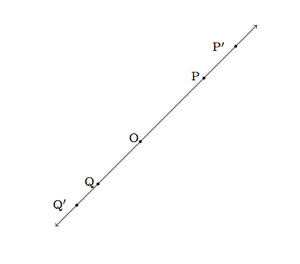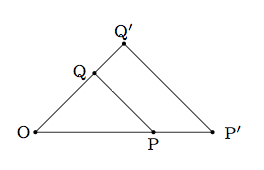# Dilations and Distances

Alignments to Content Standards: G-CO.A.2

Let $f$ be the map which dilates the plane by a factor $r \gt 0$ with repsect to a center $O$. We will denote tthe image $f(A)$ of a point $A$ by $A^\prime$.

1. Suppose $O$, $P$, and $Q$ are collinear. What is the relationship between $|P^\prime Q^\prime |$ and $|PQ|$? Draw a picture and explain.
2. Suppose $O$, $P$, and $Q$ are not collinear. What is the relationship between $|P^\prime Q^\prime |$ and $|PQ|$? Draw a picture and explain.

## IM Commentary

The goal of this task is to study the impact of dilations on distances between points. For a pair of points which are collinear with the center of the dilation, the impact of the dilation on this distance can be derived from the definition of dilations. For a pair of points which are not collinear with the center of the dilation, it is essential to use the fact that dilations map triangles to a similar triangles. Unlike rigid motions of the plane, dilations by a factor other than $r = 1$ change distances. When $r \lt 1$ points become closer by a factor of $r$ and when $r \gt 1$ they become further away by the same factor of $r$. Dilations preserve line segments so the teacher may wish to explain that $\overline{P^\prime Q^\prime}$ is the same as $f(\overline{PQ})$ so the $^\prime$ notation denotes the function $f$ throughout the problem. If students struggle to get started on this task, the teacher may wish to help them set up sample pictures and make sure that their pictures are accurately labelled. On the other hand, if the teacher desires a more challenging version of this task, she may simply give two points $P$ and $Q$, with no restrictions, and ask students how $|P^\prime Q^\prime|$ relates to $|PQ|$: the teacher will need to watch carefully and make sure that students deal with all cases.

The reasoning in part (b) of the task assumes familiarity with the standard G-SRT.2, part of which reads ''explain using similarity transformations the meaning of similarity for triangles as the equality of all corresponding pairs of angles and the proportionality of all corresponding pairs of sides.'' The standard 8.G.4 defines similarity in terms of transformations and then the relationship with the classical notion of congruent angles and proportional sides used in this task is developed in G-SRT.2.

The main standard of mathematical practice which this task illustrates is MP1, Make Sense of Problems and Persevere in Solving Them. Students must make sense of the meaning of dilations, grasp the function notation for the dilation, and reason about the effects of the dilation on lines and triangles. This is a challenging task which should be done in the classroom with ample time and with students working in groups. It is also essential, before working on this task, that students have a basic familiarity with similar triangles.

## Solution

1. We begin with a picture which we will use both to help visualize and to help solve the problem.It is also possible that $P$ and $Q$ are on the same side of $O$. One other possibility is that $P$ or $Q$ could equal $O$. The arguments in these other cases are not different in an important way, however, so we just treat the case represented in the picture. In the picture, $r \gt 1$ because $P^\prime$ is further from $O$ than $P$ and $Q^\prime$ is further from $O$ than $Q$.

The dilation $f$ maps $O$ to $O$ and changes distances on a line through $O$ by a factor of $r$. This means that $|P^\prime O| = r|PO|$ and $|Q^\prime O| = r|QO|$. In our case we have $|P^\prime Q^\prime| = |P^\prime O| + |OQ^\prime |$. Putting this together gives $$|P^\prime Q^\prime | = r|PO| + r|QO| = r|PQ|.$$ So the distance between $P$ and $Q$ is multiplied by a factor of $r$.

2. As in the part (a) we begin with a picture representing the points $O, P, Q,$ as well as $P^\prime$ and $Q^\prime$:Since $O, P,$ and $Q$ are not collinear, we cannot have $O = P$ or $O = Q$ so our picture in this case does not eliminate special cases. Since dilation with center $O$ and scale factor $r$ is a similarity transformation $\triangle OPQ$ is similar to $\triangle OP^\prime Q^\prime$. We conclude that $$\frac{|P^\prime Q^\prime |}{|PQ|} = \frac{|P^\prime O|}{|PO|} = r$$ with the last equality following from the definition of dilation by a scale factor of $r$. So the distance between $P$ and $Q$ is scaled by a factor of $r$.